﻿ Multilevel Modeling of Surface Water Quality Data in Sri LankaPublications are Open
Access in this journal
Article Versions
Export Article
• Normal Style
• MLA Style
• APA Style
• Chicago Style
Research Article
Open Access Peer-reviewed

### Multilevel Modeling of Surface Water Quality Data in Sri Lanka

American Journal of Applied Mathematics and Statistics. 2018, 6(4), 158-169. DOI: 10.12691/ajams-6-4-6
Received July 03, 2018; Revised August 05, 2018; Accepted August 19, 2018

### Abstract

Besides climate change impacts on water availability and hydrological risks, the consequences on water quality is just beginning to be studied. This research concerns the impacts of climate change on surface water quality through multilevel analysis. Multilevel modeling is a relatively new statistical technique in environmental science research, although its roots can be traced back to several other fields. The objective of this study was to evaluate the surface water quality, its spatial variation and its dependence on climatic parameters. The water quality data for seven parameters, namely Color, Turbidity, pH, Electrical Conductivity, Chloride, Total Alkalinity and Total Hardness collected from 2012 to 2014 from 68 locations around Sri Lanka was used for the analysis. These monthly water quality measurements had been made on two occasions nested within locations within districts and thus had a multilevel structure. Hence a multilevel regression model was adopted using the Bayesian Markov Chain Monte Carlo method. Since, neither of the 95% credible intervals for chemical composition (0.682, 4.945) and physical composition (0.203, 0.485) of water included the value zero, district level variances are significant. The chemical composition of water varies more with the districts compared to the physical composition of water. Several locations in Anuradhapura and Monaragala districts contributed to this significant difference in chemical composition and several locations in Ampara district presented a significant contribution to the difference in the physical composition as shown by the non-inclusion of the value zero in their individual 95% confidence bands. Further, it was observed that rain (P<0.01), temperature (P<0.01) and humidity (P<0.05) have an impact on both the chemical and physical composition of surface water. Source type (P<0.01) has an impact only on physical composition of water. The main conclusion of the study was that drinking water quality varied geographically and over time according to climatic conditions.

### 1. Introduction

The world is facing a serious problem of natural resource scarcity, especially that of water with a rapid growth of population and economic development. All known forms of life need water for their existence. The quality of water is usually described according to its physical, chemical and biological characteristics. But most of the freshwater bodies all over the world are getting polluted, thus portability of pure water is decreasing regularly. Water pollution occurs when a water body is adversely affected due to the addition of large amounts of waste materials. When harmful materials are released directly into a water body, water pollution occurs as a point source. A nonpoint source carries pollutants to the water body indirectly through environmental changes. As an instance, the nonpoint source of water pollution happens when fertilizer used in cultivation is carried into a water body by rain. Climate change influences are a key area of concern, especially when water is the underlying subject. Uncertainty about the potential climate change is a factor which impacts the water quality. The changes to precipitation and its pattern, changes to soil moisture due to temperature variations and changes to quantities evaporated from irrigated lands and irrigation reservoirs have been reasonably recognized as climate changes. Projected changes in rainfall and air temperature could affect river flows. Then the mobility and dilution of contaminants could happen. Moreover, high temperatures will affect chemical reaction kinetics 1.

Data for this study were obtained from the National Water Supply and Drainage Board and Meteorological Department of Sri Lanka. It consists of monthly surface water quality details of 68 island-wide water intake locations and monthly mean rainfall (mm), mean temperature (°C) and mean humidity (%) covering selected districts during the period 2012-2014. There are seven response variables of interest named Color, Turbidity, pH, Electrical Conductivity, Chloride, Total Alkalinity and Total Hardness. The study was limited to thirteen (13) districts in Sri Lanka due to the unavailability of data from the other districts.

The data used in the study were gathered across three main levels. Monthly water quality measurements are clustered within the locations. Locations make the level-2 units. Further, locations are grouped across the district and level-3 units can be considered as districts. There are a number of parameters which explain water quality collectively on different dimensions. Hence, methods for statistical analysis of such data should be under the umbrella of multilevel statistical methods.

Furthermore, water quality measurements are taken from individual water bodies where water bodies are located in different districts. Therefore, it can be said that water bodies are grouped within each district. Hence the level of the water quality would depend on the attributes of different climate zones as well as other factors. Rather than either taking all of the data together or considering these separately, taking a hierarchical structure is more effective for data modeling. Analyzing hierarchical or clustered structured data using traditional methods have many problems. However, the multilevel analysis allows group-wise characteristics of such data to be included in models accounting for individual behavior.

Table 1 presents the variables, their notations, respective categories and coding mechanism for preliminary analysis. Since there is a lack of methods for handling continuous data under the multilevel structure, categorized variables will be used in preliminary analysis as shown in Table 1. However, the advanced analysis will be carried out with some variables in their raw form.

All water quality variables were categorized into two categories taking the maximum desirable limit of drinking water as a benchmark. The maximum desirable limit of certain drinking water parameter was taken from SLS 722. Discretization can be more effective when continuous data are divided into three categories than a binary split 2. Furthermore, if the distribution of predictor variable has a short tail such as a uniform or normal distribution, dividing (1/3)rd split based on percentile is recommended and if the distribution shows long tail (i.e. Skewed), dividing (1/3)rd split based on lower and upper quantile is recommended through their simulation study. In order to categorize climatological variables, distribution of rainfall, temperature, and humidity were identified by drawing histograms for each variable.

### 2. Methods and Materials

2.1. Univariate Analysis Using Zhang and Boos Test

In order to identify the nature and the strength of the relationships between response variables and explanatory variables, it is essential to do a univariate analysis before going on to the advanced analysis phase. However, the usual Pearson Chi-square test fails to assess relationships accurately within the multilevel framework. The Generalized Cochran Mantel Haenszel test proposed by 3 can be used for correlated categorical data in order to assess the initial relationships among response variables and explanatory variables in a multilevel framework. It provides three different kinds of test statistics, namely TEL, TP and TU. Previous simulation studies 3 have proved that TP is the preferred test statistic over TU and TEL as when there are a small number of strata as in the dataset of interest.

2.2. Principal Component Analysis

Principal component analysis (PCA) is a multivariate technique which involves a mathematical procedure that converts a set of correlated response variables into a smaller set of uncorrelated variables called principal components (PCs). This technique is usually appropriate when the variables are highly correlated. A few artificially created linear combinations from the PCA, are used to explain the total variability of the data. When performing a PCA one needs to determine the actual dimensionality of the space in to which data fall. This is given by the number of eigenvalues that are not zero (or not close to zero). The eigenvector associated with the largest eigenvalues has the same direction as the first principal component. The direction of the second principal component is determined by the eigenvector which is associated with the second largest eigenvalue. The eigenvalues greater than one are selected since these account for most variance of data.

2.3. Univariate Multilevel Linear Regression Model
2.3.1. Single Level Regression Model

Suppose there is only a single explanatory variablefor simplicity. A general model for a single normally distributed response is defined as follows,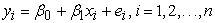(1)

Assume that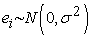Where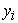is the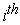value of the response variable Y,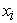is thevalue of the predictor variable X and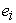is the error in the approximation ofHere,is called the slope and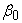is called the constant coefficient or the intercept and these are the parameters in the model.

2.3.2. Standard Regression Assumption

For the purpose of inference or prediction using linear regression models, there are four principal assumptions which should be satisfied. These are the linearity and the additivity of the relationship between the response Y and the predictors, the normality of the error distribution, statistical independence of the errors and homoscedasticity of the errors.

2.3.3. Multilevel Regression Model

Here, the single level model extends to the multilevel model to allow the second level variation on the response variable. Therefore, it is considered by the random intercept or variance component model which allows the response variable to vary with the level- 2.

Suppose there exists a single explanatory variable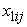measured at the individual level, then (1) is extended to a two-level random intercept model as,(2)In here, the intercept consists of two terms as a fixed componentand the level 2 specific component, the random effect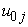.

Assume,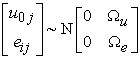(3)

Where,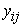is the dependent variable measured forlevel 1 unit nested within thelevel 2 unit,is the value of the level 1 predictor,is the random error associated with thelevel 1 unit nested within thelevel 2 unit,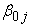is the random intercept of the model,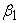is the regression coefficient associated with X for the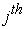level 2 unit.

In general, wherever an item has two subscripts ij, it varies at both level 1 and level 2. Multilevel linear regression models also depend on the assumptions which are mentioned in single-level linear regression models previously. However, they may be modified for the multilevel scenario. The two-level model can easily be extended to higher levels.

2.3.4. Variable Selection and Model Comparison

The process of the variable selection determines the best subset of predictors which explain the response well. Backward Elimination Procedure, along with the Wald statistic and Deviance Information Criteria (DIC) was used to select the predictors. MLwiN uses Iterative Generalized Least Squares (IGLS) method to estimate the model parameters. It uses likelihood based frequentist methods. Due to this reason, models cannot be compared using the usual likelihood ratio test. Model comparison was based on the Deviance Information Criterion (DIC) value which is a powerful tool to compare models. In order to get the DIC value for each step, the Residual Iterative Generalized Least Squares (RIGLS) procedure is followed by Markov Chain Monte Carlo (MCMC) method. Further, the model selection procedure was implemented again under the robust method 4 in order to get more reliable results when the model assumptions are not satisfied. Moreover, robust methods are more reliable as these rely less on the underlying model assumption. MLwiN software facilitates implementation of the robust standard error for fixed and random parts of the model.

2.3.5. Residual Analysis and Model Adequacy

After fitting a model it is essential to carry out a model diagnostics process in order to determine whether all the underlying assumptions are valid and the fitted model is adequate or not. Otherwise, invalid inferences may be made. In the multilevel structure, the residual analysis is much more complicated than in the classical approach. Moreover, it is important to note that the residual analysis and diagnostic testing of multilevel models is less well known. Even though the specification of the multilevel model differs with respect to the nature of the response variable, the theory behind the diagnostic testing is the same. Unlike other models, the multilevel models have residuals at each level. When the levels are increased, computations, as well as analysis of the residuals at different levels, become more difficult. 4 pointed out that, the higher level residuals are more important than the lower level residuals for the residuals analysis since, the sample size of the higher levels is relatively smaller than the lower level.

2.4. Bayesian Inference and Markov Chain Monte Carlo (MCMC) Method

While the frequentist approach makes population-based inferences only from the sample data, the Bayesian approach uses prior information along with the sample data to make inferences. Furthermore, frequentist inference assumes that the parameters of interest are a fixed constant. The flavor of Bayesian inference is the assumption that nature can be represented by the model of a probability distribution. Therefore, the Bayesian approach differs from the frequentist approach and it is sequential in nature.

Markov Chain Monte Carlo (MCMC) methods are the estimation techniques which use Markov Chains for sampling from a probability distribution. These methods can be used for both frequentist and Bayesian inference. However, MCMC methods are more common for the Bayesian framework and MLwiN also uses the MCMC procedure for Bayesian modelling. The Bayesian approach produces a posterior distribution to make inference using a prior distribution and a likelihood function of sample data with the distributional assumption. However, it is difficult to find the implicit form of the posterior distribution practically and it requires high computational power. MCMC methods give a solution to this problem following a simulation-based procedure. These have the ability to sample repeatedly each and every sample depending on the previous one, from any desired distribution. Monte Carlo integration calculates an expectation by averaging the Markov Chain samples. Since obtaining the joint posterior distribution directly is difficult, MCMC methods use conditional posterior distributions for unknown parameters as an alternative approach 5.

2.5. Robust Methods for Multilevel Analysis

As in the other regression models, residuals play an important role in the model diagnostic process in multilevel modelling this is also the case. The assumptions underlying the distribution are always tested through the residuals. In general, the individual observations are not independent in the multilevel context. They have some dependencies within the clusters. Therefore, the errors cannot be assumed to be independent and identically distributed (i.i.d). Moreover, the sample sizes at the highest levels are by definition smaller than the sample sizes at the lowest level. Detection of outliers is also more difficult in the hierarchical structure 6. All of these reasons may lead to the violation of the assumption of normally distributed residuals. When the assumption of normality is not met, standard errors can be biased. Simulation studies by 6 suggest that only the standard errors of the random effects at the higher levels can be highly inaccurate if the normality assumptions at higher levels are not satisfied. However, the regression coefficients and their standard errors show little or no bias. One method of overcoming this problem is to correct the asymptotic standard errors when the underlying distributional assumption does not hold. One well-known correction method is to use robust standard errors.

Robust methods are statistical methods for the estimation and the establishment of confidence intervals that are not very sensitive to violation of the assumption of the underlying statistical 7. For this purpose, robust methods which use the sandwich or Huber/White estimator of the standard error can be used. Furthermore, these corrected standard errors are called robust standard errors. These are available in several software packages which have the facility of multilevel analysis. MLwiN has an option to use, robust sandwich estimators for the standard errors of the variance components. These use the observed residuals to estimate the variance components in the model.

2.5.1. Sandwich Estimators

The usual estimator of the sampling variance and covariances in the maximum likelihood approach is the inverse of the Information matrix. The asymptotic variance co-variance matrix of the estimated coefficients can be defined as,Where,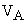is the asymptotic covariance matrix of the regression coefficients and H is the Hessian matrix.

The sandwich estimator is given by,Where,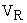is the robust covariance matrix and C is a correction matrix which is sandwiched between the twomatrices and it is based on the observed raw residuals. If the residuals follow a normal distribution,andare both consistent estimates of the covariance of the regression coefficients. However, the model based asymptotic covariance matrixis more efficient. If the residuals cannot be assumed to be normal, robust standard errors better reflect with the results while asymptotic standard errors tend to be biased 3.

### 3. Results

With the presence of the hierarchical nature of the dataset of interest, the most commonly used tests such as the chi-square test cannot be performed here. Hence, the GCMH test which is proposed for correlated categorical data was carried out under the univariate analysis. According to the structure of the data, the water quality measurements and all the explanatory variables are measured at the location level, monthly. Thus location and district can be considered as the second and third levels respectively. Hence, the univariate analysis was performed considering combinations of the district and location as a respective stratification factor. The test carried out was the GCMH test taking one response variable at a time for each explanatory variable. The results are given in Table 2.

The 20% for the significance was considered as the liberal level at this univariate stage. The Generalized CMH results indicated that the rain has a significant impact on all the chemical as well as the physical water quality parameters. The source type has shown a significant impact on most of the chemical parameters except Cl of water. Only the color of the water depicts a significant association with source type when considering the physical parameters. The humidity has a significant impact on most of the chemical as well as physical parameters except EC of the water. It indicates that the temperature has a significant impact only on the alkalinity and color of the water, not on others.

Since there are 7 correlated response variables, it leads to a more complex situation in the modeling phase. In order to overcome this, principal component analysis (PCA) which is a dimension reduction technique, was used to decompose the response variables into several sets. Since different parameters are measured in different units, the correlation matrix was used to perform PCA. The first principal component explains 58% of the total sample variance and the second component explains 28.8% individually. Hence, the first two principal components collectively explain 86.8% of the total sample variance. The eigenvalues indicate that the two components provide a reasonable summary of the data, accounting for about 86.8% of the total variance. Subsequent components, each contributes about 9.5% or less. Consequently, sample variation is summarized very well by the first two principal components. The first component has equally large positive loadings on all the chemical quality parameters. This suggests that the first component is primarily a measure of water quality chemically. The second component has high positive loadings on all the physical quality components. This suggests that the second component is primarily a measure of water quality physically.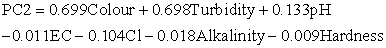From this point, the whole analysis was done separately for physical and chemical water quality parameters as suggested by the PC’s.

3.1. Fitting a Multilevel Regression Model

The advanced analysis basically focuses on the score values derived from the Principal Component Analysis. Initially, three-level linear regression models were fitted to the score values of the first and second principal components separately. The first level consists of the monthly water quality measurements, the second level consists of the locations and the third level consists of the districts. The model building procedure was carried out using MLwiN v2.19. In order to get the DIC values which can be used for model selection 8 the Markov Chain Monte Carlo (MCMC) method was implemented with a burn-in of 200 and a chain length of 5000. The MCMC method for each model was used after convergence with Restrictive Iteration Generalized Least Squares (RIGLS) method.

All climatological variables were taken in their continuous form since the discretization of continuous variables may cause the loss of power. However, due to some non-convergence problems which arose with the “Rain” variable, its natural logarithm was used for the model building process.

Two univariate multilevel models for the first and second principal components which explain the chemical and physical water quality respectively were fitted following the backward elimination procedure. Model fitting was carried out stepwise starting from the model with all main effects and all two-way interaction terms. MLwiN could not fit the full model which has all the main effects and all possible higher order interactions due to non-convergence. In order to determine the most non-significant variable in the model, the Wald statistic together with the DIC value was used at each stage in the model building procedure. Furthermore, MLwiN v 2.19 takes the lowest category as the base with the presence of the categorical variables.

For example, the Table 3 shows that the base level of “Source” variable which is one of the factors in this study, is running water.

3.1.1. The Multilevel Regression Model for the First Principal Component Which is Related to the Chemical Water Quality

The score values of the first principal component were used as the response variable to model the chemical water quality. Only main effects and two-factor interactions were considered in the initial stage as MLwiN crashed when fitting the full model with all possible interactions. In order to identify the most non-significant variable, the P-value of the Wald statistic for each variable was tested at the 5% level of significance. With the best-fitted model, it is essential to check the suitability of the multilevel concept by checking the significance of the level 2 (Location) and the level 3 (District) variance by the following hypothesis.

Ho: Unexplained level i variance is zero

H1: Unexplained level i variance is not zero.

Since the value zero does not lie within the 95% confidence intervals ([0.888, 1.969] and [0.682, 4.945]), both location level and district level variance are significant respectively implying that of the suitability of the multilevel approach.

In multilevel modeling, the requirement of adequacy tests applies most forcefully to the highest levels, since these generally have the smaller sample size 3. Initially, Normal probability plots were drawn for level 2 and level 3 and Anderson Darling test was also performed to check the normality. Even though district level residuals satisfy the assumption of normality, location level residuals do not satisfy the normality as seen from both the normal probability plot and the Anderson Darling test. The problem of non-normality in residuals usually occurs in the practice of multilevel modeling. However, 3 recommend two approaches to address the violation of the normality assumption in the multilevel regression model with the discussion of their strengths and weaknesses. These two methods are the use of robust standard errors and Bootstrapping. Furthermore, they explain that only the standard errors for the random effects at the higher level are highly inaccurate if the distributional assumptions concerning the higher level errors are not fulfilled. Robust standard errors turn out to be more reliable than the asymptotic standard errors. The robust standard errors were used to refit the model as it is one of the recommended approaches and MLwiN also provides the facility to use robust standard errors for the parameter estimation in the model. The model selection procedure was performed again applying the robust standard errors.

The robust standard errors were equal or very close to the asymptotic standard errors, except for the random variance in all the levels. However, the robust standard errors do not completely correct this, but they do result in more accurate significance tests and confidence intervals. However, there were no significant differences between the estimates in the two models and it was decided to go with the model under robust standard errors. Therefore, the fitted model under the robust method was considered as the final model for the scores of the first principal component to explain the chemical component in the water quality. PC1 is assumed to follow a Normal distribution and is denoted byWhere, XB is the fixed part of the model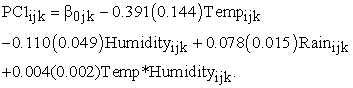3.1.2. Residual Analysis of the Final Model for PC1

After fitting the model, the model adequacy was checked by using the Anderson Darling test, Normal probability plots, and Caterpillar plots. Since the P-values for the Anderson Darling test statistic for district and location level residual are more than 0.05, the district and location level residuals satisfy the assumption of normality.

• Figure 1. Caterpillar plot for level 3 residuals
• Figure 2. Caterpillar plot for level 2 residuals
• Figure 3. Significant Locations with positive deviation for 1st PC

The Caterpillar plot for level 3 residuals in Figure 1 depicts that five residuals do not contain zeros in their 95% confidence bands and Caterpillar plot for level 2 residuals in Figure 2 shows that 14 residuals do not contain zeros in their 95% confidence bands. These imply a significant difference from the overall mean predicted by the fixed part from the model. Moreover, it can be seen that some locations show a negative residual deviation while others show positive deviations.

Figure 3 and Figure 4 depict the locations which have a positive and negative deviation from the overall mean predicted by the fixed part of the model respectively. Figure 3 indicates locations within districts that show a higher chemical properties compared to the average. Figure 4 shows locations within districts that show lower chemical properties than the average.

• Figure 4. Significant Locations with negative deviation for 1st PC

3.1.3. Interpretation and Calculation of the Parameter Estimates

Effects of Rain on the Response variable

As “Rain” is a continuous variable, 0.078 represents the difference in the predicted value of Y which is the score of the first PC for each one unit difference in logarithm value of the “Rain”, if others variables are held constant. This means that if the log of “Rain” is increased by one unit and others do not change, PCA 1 will differ by 0.078, on average. For the regression coefficient for the log of “Rain”, the 95% confidence interval runs from 0.0486 to 0.1074.

Effects of Temp*Humidity on the Response variable

When the interaction terms are significant, the change in response varies for each level or value of the variables in the interaction term. Therefore, it was decided to interpret interaction terms by using the following calculations.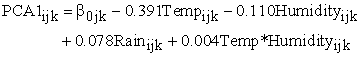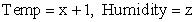(1)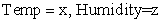(2)When humidity remain at its average value (z = 80.9) and other variables remain as unchanged while temperature increases from x to x+1 then there is a decrease of 0.067 for PC1.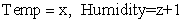(1)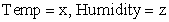(2)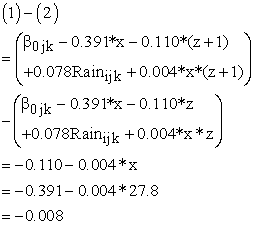When temperature remains at its average value (x = 27.8) and remains unchanged while humidity increases from z to z+1 then there is a decrease of 0.008 for PC1.

Using the same argument, when temperature increases from x to x+1 and humidity increases from z to z+1 when x and z are both on average then there is a decrease of 0.00622.

3.1.4. Multilevel Regression Model for the Second Principal Component which Relates to the Physical Component of Water Quality

The score values of the second principal component were used as the response variable to model the physical component of water quality. Similarly, the above model building process was followed for the second principal component. P-value of the Wald statistic for each variable was tested at 5% level of significance to determine the significance of the estimates at each stage. After the final model was fitted, the suitability of the multilevel concept was checked by testing the significance of the level 2 and level 3 variance.

Ho: Unexplained level i variance is zero

H1: Unexplained level i variance is not zero

As the value zero does not lie within the 95% confidence intervals ([0.010, 0.453] and [0.203, 0.485]), both location level and district level variance are significant respectively implying that of the suitability of the multilevel approach.

Priori concluding the fitted model is adequate, it is important to check the assumptions underlying the regression model. Therefore, as the first step normality of the level 2 and level 3 residuals were examined by drawing normal plot as well as performing Anderson Darling tests.

As in the previous case which is the model for PC1, level 2 residuals violate the normality assumption even though level 3 residuals follow the normal distribution. Therefore, it was decided to refit the model again estimating the parameters using robust standard error as previously done. The backward elimination method was adopted for the model selection procedure. The significance of the parameter wholly depends on the Wald statistic. The fitted model for PC2 is given below.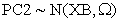Where, XB is the fixed part of the model.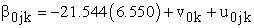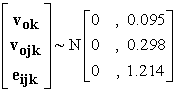3.1.5. Residual Analysis of the Final Model for PC2

Similarly, the model adequacy was checked by using the Anderson Darling test, Normal probability plots, and Caterpillar plots. The district and location level residuals satisfy the assumption of normality as the P-values for the Anderson Darling test statistic for district and location level residual are more than 0.05.

Caterpillar plot depicts that three residuals do not contain zeros in their 95% confidence bands. These districts are Ampara, Hambantota, and Kegalle. They imply significant differences from the overall mean predicted by the fixed part from the model.

Furthermore, it can be seen that Ampara and Hambantota districts show negative residual deviation while Kegalle district presents positive residual deviation according to the Figure 5. Therefore, these districts show a high district effect on the second principal component.

In Figure 6, it is interesting to see that there are 11 water intake locations that exhibit 95% confidence bands that do not include zero. Furthermore, it implies that 4 locations have positive deviations, and seven locations have a negative deviation from the overall mean predicted by the fixed part of the model. Thus, these locations give a high contribution to the location effect of the model.

• Figure 5. Caterpillar plot of level 3 residuals
• Figure 6. Caterpillar plot of level 2 residuals
• Figure 7. Significant Locations for 2nd PC

This indicates that the 4 locations in Kegalle have a higher physical component compared to the average and the 7 locations in Ampara and Hambantota have a lower physical component compared to the average.

3.1.6. Interpretation and Calculation of the Parameter Estimates

The intercept-only model estimates the intercept as -21.5454, which is simply the average score value of PCA2 across all locations and districts.

Effects of Temp*Humidity on the Response variable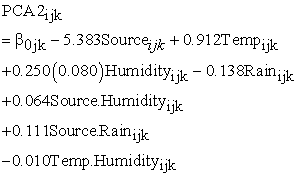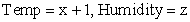(1)(2)When humidity remain at its average value (z = 80.9) and other variables remain as unchanged while temperature increases from x to x+1 then there is an increase of 0.103 for PC2.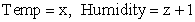(1)(2)Base category of the Source variable is “Running”. Its value is 1 which is defined in the introduction chapter. Therefore, it takes value of one.There is an increase of 0.04 units for PC2 when humidity is increased by one unit while temperature remains at its average (27.48), Source variable is at its base level (running water) and all other variables remain the same irrespective of their values.

Effects of Source*Humidity on the Response variable

This interaction term can be interpreted while considering the base category (running water) of the Source variable. It takes the value of one. Calculations are same as above.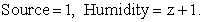There is an increase of 0.039 units for PC2 when humidity is increased by one unit while temperature remains at its average (27.48), Source variable is at its base level (running water) and all other variables remain the same irrespective of their values.

Effects of Source*Rain on the Response variableThere is an increase of 0.249 units for PC2 when logarithmic value of the rain is increased by one unit while Source variable is at its base level (running water) and all other variables remain the same irrespective of their values.

When the Source variable is not at its base level, that’s standing water, same variables which predict PC1 are effected to the PC2 also. The running water more related with physical component of the water than the standing water source. As there are three interactions in this model it is tedious to calculate the scenario of x+1, z+1 compared with x, z. However, using the same principles as in the case of PC1 this can be achieved.

### 4. Discussion

The temperature, Humidity, Rain and the interaction effect Temperature*Humidity showed significance in the model for the first principal component. The 1st PC is composed of pH, EC, Cl, Alkalinity, and Hardness of the water. The Rain which was significant with all these chemical water quality variables in the univariate analysis was also significant in the advanced analysis phase. However, Source type was significant with most of the chemical water quality variables, it fails to be significant in the advanced analysis.

The Source Type, Temperature, Humidity, Rain and the interaction effect Source*Humidity, Source*Rain and Temperature*Humidity, showed significance in the model for the second principal component. The 2ndPC is composited of Color and Turbidity of the water. In the univariate analysis, while all the covariates were significant with the color of the water, only Rain and Humidity were significant with Turbidity of water. However, all the covariates were significant with the physical component of the surface water.

Since the rain was significant in both chemical and physical components of the surface water, it can be concluded that the rain has an impact on the surface water quality. Past evidence is also available to prove this relationship. A study conducted in South Korea has shown that the amounts of the rainfall or patterns of rainfall event have an impact on changes of water quality 9.

It can be concluded that the temperature has an impact on the surface water quality since it was significant in both models. Moreover, when looking at the available literature, there is a study in France which discusses climate change on water quality. The study revealed that the relationship between temperature and water quality further concluding stream temperature changes could have occurred approximately due to air temperature changes 10

Furthermore, when considering 3rd level variation for the chemical and physical component of water, it can be seen that the chemical component varies most with the districts compared to the physical component. However, both chemical and physical components vary with water intake locations in the same way.

4.1. Limitations of the Study

In the advanced model building phase, MLwiN crashed with the 3 level multivariate multilevel model for both physical and chemical parameters. Therefore, two univariate multilevel models were fitted for both first and second principal components which explain the chemical and physical component of water respectively.

Non-convergence of the model occurred with the “Rain” variable and MLwiN crashed. Therefore, the natural logarithm of “Rain” was used instead of “Rain” variable in both the model building process.

### 5. Conclusion

The conclusion of the study was that water quality varied geographically and over time according to climatic conditions. Furthermore, higher consideration should also be given to climatic factors such as rain, temperature, and humidity to improve water quality.

### Acknowledgements

I would like to extend my sincere thanks to all the lecturers of the Department of Statistics, University of Colombo supporting in numerous ways.

### References

  Whitehead, P. G., Wilby, R. L., Battarbee, R. W., Kernan, M., & Wade, A. J. (2015). A review of the potential impacts of climate change on surface water quality A review of the potential impacts of climate change on surface water quality, 6667 (November). In article View Article  Gelman, A., & Park, D. K. (2009). Splitting a Predictor at the Upper Quarter or Third and the Lower Quarter or Third. The American Statistician, 63(1), 1-8. In article View Article  Maas, C. J. M., & Hox, J. J. (2004). Robustness issues in multilevel regression analysis. Statistica Neerlandica, 58(2), 127-137. In article View Article  Goldstein, H. (1999). Multilevel Statistical Models, London. In article View Article  Browne, W. J. (2009). MCMC Estimation in MLwiN v2.10. Center for Multilevel Modeling, University of Bristol. In article  Maas, C. J. M., & Hox, J. J. (2004). The influence of violations of assumptions on multilevel parameter estimates and their standard errors. Computational Statistics & Data Analysis, 46(3), 427-440. In article View Article  The SAGE Handbook of Multilevel Modeling. (2013). SAGE Publications. Retrieved from https://books.google.com/books?id=_Y1SAAAAQBAJ&pgis=1. In article View Article  Browne, W. J. (2004). An illustration of the use of reparameterisation methods for improving MCMC efficiency in crossed random effect models. Multilevel Modelling Newsletter, 16, 13-25. In article View Article  Bates, B.C., et al., (2008). Climate change and water. Geneva: IPCC Technical paper. In article  Ducharne, A. (2008). Importance of stream temperature to climate change impact on water quality, 797-810. In article View Article  Hox, J. J., & Maas, C. J. M. (2005). Multilevel Analysis. Encyclopedia of Social Measurement (Vol. 2). In article View Article  Zhang, J., & Boos, D. D. (1997). Mantel-Haenszel test statistics for correlated binary data. Biometrics, 1185-1198. In article View Article  PubMedThis work is licensed under a Creative Commons Attribution 4.0 International License. To view a copy of this license, visit http://creativecommons.org/licenses/by/4.0/